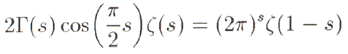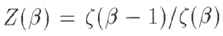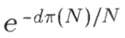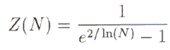## "Applications of Statistical Mechanics in Prime Number Theory"

Marek Wolf

[This is a summary of a 1999 preprint. It's a review article based on a lecture he gave in Budapest.
An article with the same title was published in Physica A in 1999.]

## 1. Introduction

Wolf begins by briefly referring to the general phenomenon of the "number theory–physics crossover". Two references to the application of prime number theory to quantum chaology in particular are given [1,2,3]. Two mathematical properties which are normally associated with physical systems have been detected in the distribution of prime numbers by Wolf himself, namely multifractality  and 1/f noise . A paper which numerically calculates Lyapunov exponents (familiar to chaos theorists) for the distribution of primes is mentioned . Finally an article is cited  wherein the authors apply the Wiener–Khintchine formula, which relates spectral densities and the autocorrelation function, to the problem of the distribution of pairs of primes {p,p+2}.

## 2. The Riemann Hypothesis and statistical mechanics

Wolf describes the Riemann Hypothesis as "probably the most famous open problem in mathematics". The Riemann zeta function is defined, and its zeros in the complex plane are briefly described, leading to a statement of the RH itself. The functional equationis stated. Recall that the zeta function is initially defined for complex s with Re(s) greater than 1, by means of a simple infinite series. The functional equation allows the definition to be extended to the other half of the complex plane. It turns out that this equation is analogous to the Kramers–Wannier duality relation  for the partition function of the 2-dimensional Ising model. This suggests that the zeta function is in some sense acting as a partition function, which is the primary quantity in statistical mechanics. Two papers are mentioned which examine this analogy. In  Bernard Julia defines an abstract numerical "gas" whose partition function is the zeta function. In  A. Knauf finds a spin system whose partition function is the ratioLee–Yang  type theorems can be used to localise the zeros of partition functions, and the Riemann Hypothesis concerns the restriction of the zeros of the zeta function. Hence an interpretation of the zeta function as a partition function opens up the possibility of applying Lee–Yang theorems to the problem of proving the RH.

Another approach to proving the RH is the spectral interpretation. The central idea is that if a Hermitian operator on a Hilbert space can be found whose eigenvalues correspond to the imaginary parts (the "heights") of the nontrivial zeros of the zeta function, then the RH follows. Equivalently, a Hermitian operator T on a Hilbert space is sought such that the zeros of zetacan be interpreted as the eigenvalues of 1/2 + i T. This idea was first proposed by Hilbert and Pólya in the early part of the century. A couple of articles approaching the proof RH from this point of view [12,13] and a review of such attempts  are cited.

## 3. Random walks and prime numbers

The earliest use of prime numbers to generate a random walk was Billigsley's employment of the Möbius function in the 1973 article "Prime Numbers and Brownian Motion" . Although no mention of it appears in this review by Wolf, I recall seeing in Mehta's book Random Matrices  a brief mention of a "novel" approach to the subject involving a kind of "Brownian motion" introduced by Dyson (I believe). This may be unrelated, but random matrix theory (in particular Gaussian Unitary Ensembles) is being used in the "spectral interpretation" attack on the RH mentioned earlier.

The Mertens conjecture that |μ(1) + . . . + μ (n)| is less than sqrt(n) (where μ is the Möbius function) is mentioned. Recall that μ is defined on the natural numbers such that μ (1) = 1, μ(n) vanishes when n is divisible by a square of a prime, and is otherwise equal to (–1)k where k is the number of prime divisors of n. The Mertens conjecture relates to a kind of random walk, and its truth would imply the truth of the RH (but the converse is not true). The conjecture was actually disproved in 1985, but a slight modification (stating that μ(1) + . . . + μ (n) does not grow faster than n1/2 + ε for any positive ε) is equivalent to the RH, and therefore obviously still stands.

Most interestingly, Wolf notes that in , the Möbius function is given a physical interpretation. Specifically, μ(n) can be interpreted as the operator (–1)F giving the number of fermions in quantum field theory. The fact that μ(n) = 0 when n is not "squarefree" in this way becomes equivalent to the Pauli exclusion principle.

Its worth pointing out that the Möbius function has a deeper connection to the distribution of primes than is immediately apparent in its definition. The reciprocal of the Riemann zeta function acts as a generating function for the Möbius function in the following sense:The right-most expression is an example of a Dirichlet series, whose coefficients are the values μ(n).

Wolf also describes a random walk he devised based on the difference between the number of twin primes (pairs of primes of the form {p,p+2}) and "cousin primes" (pairs of primes of the form {p,p+4}) which have appeared at each step of the walk .

## 4. Prime numbers as a gas of harmonic oscillators

In the previously mentioned article , B.L. Julia constructs the "free Riemann gas", an abstract thermodynamic system based on the prime numbers. It's a fairly uncontrived notion, with the primes playing the role of the "atomic" particles, each pn having energy log pn . Most importantly, its partition function is the Riemann zeta function. Wolf has constructed a variant on this – his "prime gas" also has the primes acting as particles, but in this case pn has energy pn+1 pn, i.e. the distance to the next prime.

In and earlier article , Wolf investigated the value of hN(d), which is defined very simply to be the number of pairs of consecutive primes less than N, which are separated by a gap of size d. A computer-generated histogram is included, which strongly suggests certain unexpected regularities. In particular hN(d) appears to decrease exponentially with d. A proportionality relation is given which involves the termNoting that the number hN(d) is directly related to the probability of randomly selecting a pair of consecutive primes separated by a gap of size d or in the thermodynamic interpretation, the probability of energy level d occuring), and recalling the prime number theorem π(N) ~ N/log N, Wolf points out that by identifying tempertature T with log(N), this term becomes the Boltzman factor.

He then goes on to argue that for large N the partition function of the "prime gas" becomeswhich corresponds exactly to the partition function of a harmonic oscillator with parameters ω = h-bar/4.

Wolf admits that his argument involves several approximations, and therefore states: "...we can say that the primes behave like a harmonic oscillator in the 0-th order approximation. In higher orders there are corrections which are responsible for all subtleties and mysteries in the distribution of prime numbers."

Finally, another article which relates the primes to a harmonic oscillator is mentioned . In this, the author successfully constructs a quantum potential (in the semiclassical way) whose eigenvalues, after rescaling, correspond exactly to the primes. The potential W(x) for large x corresponds to a harmonic oscillator, although apparently this derivation also involves a lot of crude approximations.

References

 M. Berry, "Some quantum-to-classical asymptotics", in Chaos and Quantum Physics, eds. A. Voros, et al. (Elsevier, 1991); R. Aurich et al./i>, "Universal signatures of quantum Chaos", Physical Review Letters 73 (1994), 1356; Quantum Chaos, CXIX "Enrico Fermi" International School of Physics, eds. G. Casati, et al. (North Holland, 1993)

 P. Sarnak, "Arithmetic quantum chaos", Israeli Mathematical Conference Proceedings 9 (1995), 183

 N. Argaman, et al., "Correlations in the actions of periodic orbits derived from quantum chaos", Physical Review Letters 71 (1993) 4326–4329; E. Bogomolny and P. Leboeuf, "Statistical properties of the zeros of zeta functions: Beyond the Riemann case", Nonlinearity 7 (1994) 1155–1167

 M. Wolf, Physica A 160 (1989), p. 24

 M. Wolf, "1/f noise in the distribution of primes", Physica A 241 (1997), p.439

 Z. Gamba, J. Hernando and L. Romanelli, "Are prime numbers regularly ordered?" Physics Letters A 145 (1990), p.106

 H. Gopalkrishna Gadiyar and R. Padma, Ramanujan–Fourier Series, The Wiener–Khintchine formula and the distribution of prime pairs, Physica A 269 (1999) 503–510

 H.A. Kramiers, G. H. Wannier, Physical Review 60 (1941) p.252

 B.L.Julia, "Statistical theory of numbers" in Number Theory and Physics, eds. J.M.Luck, et al. (Springer‐Verlag, 1990) p. 276, and Physica A 203 (1994) p.425

 A. Knauf, Journal of Statistical Physics 73 (1993) p.423; Comm. Math. Phys. 153 (1993) p.77 and 196 (1998) p.703

 T.D. Lee and C.N. Yang, Physical Review 87 (1952) p.404 and p.410

 S. Okubo, Journal of Physics A 31 (1998) 1049–1057

 A. Connes, C.R. Acad. Sci. Paris, 323 (1996) p.1231; J.-B. Bost and A. Connes, Selecta Math. (New Series) 1 (1995), 411

 P.B. Cohen, "Dedekind zeta functions and quantum statistical mechanics", preprint ESI 617 (1998)

 P. Billingsley, "Prime numbers and Brownian motion", American Mathematical Monthly 80 (1973) 1099–1115

 M.L. Mehta, Random Matrices and the Statistical Theory of Energy Levels, 2nd edition (Academic Press, 1991)

 D. Spector, Comm. Math. Physics 127 (1990) p.239

 M. Wolf, Physica A 250 (1998) p.335

 G. Mussardo, "The Quantum Mechanical Potential for the Prime Numbers", preprint ISAS/EP/97/153; see also R. Matthews, "Prime Suspects", New Scientist, 10th January 1998, p.18

archive      tutorial      mystery      new      search      home